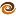# EmptyCrate

#### About Me | Contact Me | Training |ChaiScript.com | YouTube Channel

C++ templates is a huge topic that we will not fully cover here. While we have covered templates in the past, this article will cover the very basics and the reasons why we would want to use templates.

A simple case of why you would want to understand templates is wrapped up in a question I used to ask when I would interview C++ developer candidates, “write for me a max() function which can compare any two values of the same type.”

The average developer would try and figure out a method of creating a set of classes or functions which can encapsulate all the various types of objects that might want to be compared. This method would create a huge amount of complexity and did not fullfill the requirement of “any type.”

Not one developer even mentioned the classic C answer:

``````#define MAX(A,B) A>B?A:B
``````

However, since macro expansion is a text replacement, the following example presents a serious problem:

``````MAX(i++, j++)
``````

Which is compiled as:

``````i++>j++?i++:j++
``````

Also, what happens in the case where you try to compare two different types:

``````MAX("hi", 5);
``````

This would more than likely compile and not do anything at all expected.

What we need is a way to compare two values which works with any data type, is type safe and does not have the text replacement problems of a macro expansion. Also, it sure would be nice if the new method retained the performance of a macro.

The answer lies in C++ templates. Templates provide a programmer with the means of writing generic code. With a template the programmer can say: “Let the user of my library use any type as long as it can be compared to a itself with the less than operator.”

The C++ template version of the max function becomes:

``````template
const T &max(const T &f, const T &l)
{
if (f < l) return l;
return f;
}
``````

Our use of the new max function is almost as simple as the use of the last version:

``````max(5, 6);
``````

That `` it is a little cumbersome though. As it turns out the template compiler is able to automatically determine the types of template function arguments:

``````max(5,6); //Automatically generate an  version
``````

So what are we actually doing here? By creating a template function we are allowing the compiler to generate code for us! When we use the function: `max` the compiler is generating code that looks like this:

``````const int &max(const int &f, const int &l)
{
if (f < l) return l;
return f;
}
``````

All of the overhead for using templates is put up front, at compile time. No runtime overhead is added.

This code represents the simplest use of template functions and does not cover template classes at all. Templates are extremely flexible and can be used in many diverse ways. In fact, the template compiler in the C++ language is turing complete.

Templates themselves can be very complex to write and very difficult to debug. However, the resulting library’s flexibility, simplicity and code reuse will make up for the initial complexity.

The one feature notably missing from template programming is the ability to create a function with a variable number of template arguments. This feature is coming in the next version of C++ (see “variadic templates”).

By the way, an answer I would have accepted to the interview question is, “why should I write a max function, instead of just using `std::max`?” `std::max` is a standard library implementation of the template function example I gave here.

My Videos

Related